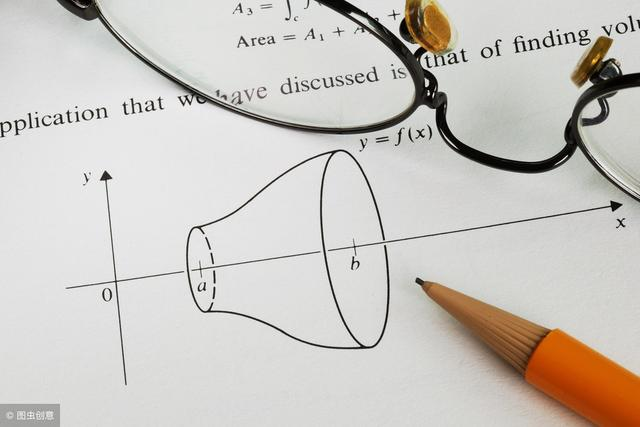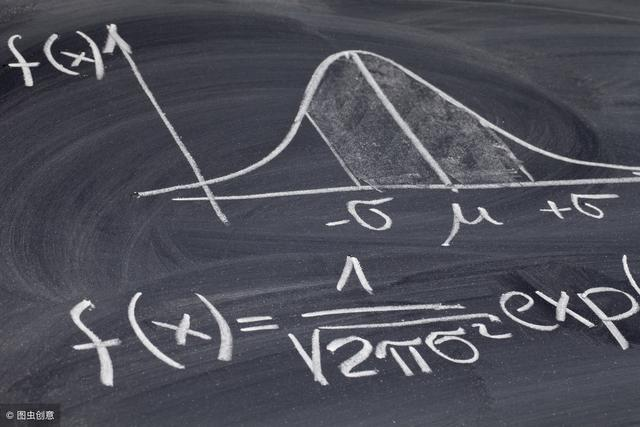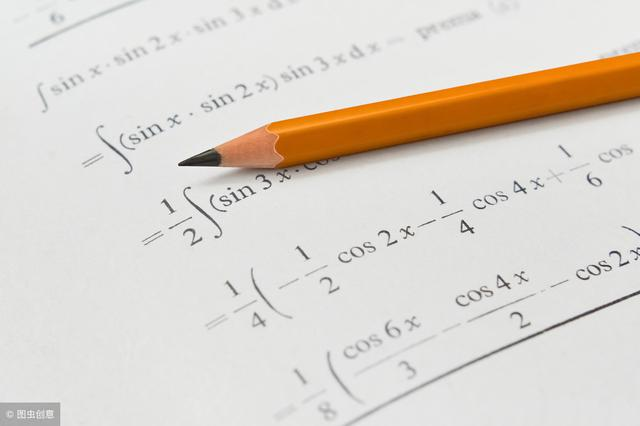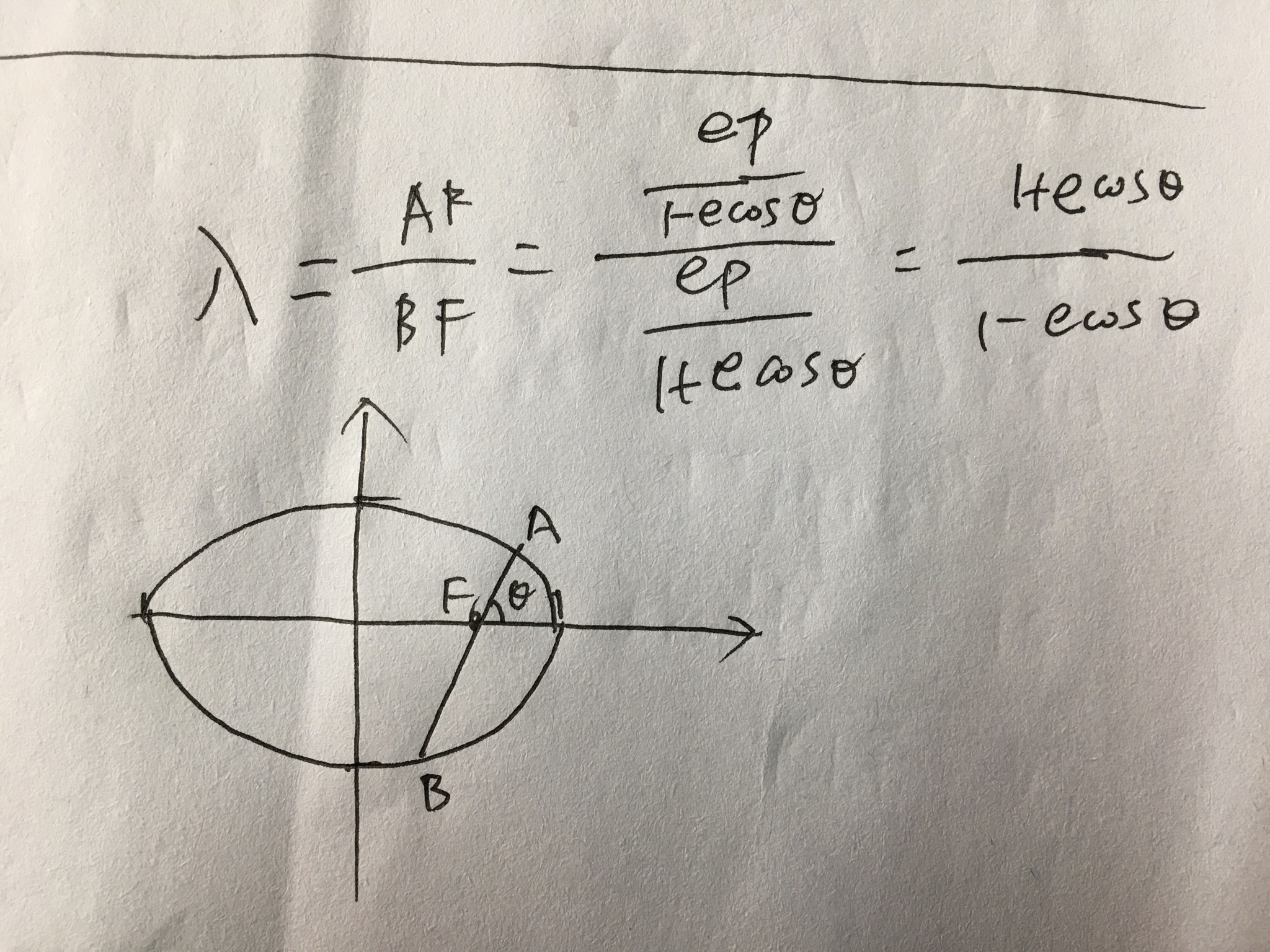• 100任意锐角的正切值等于它的余角的余切值，任意锐角的余切值等 于它的余角的正切值 101圆是定点的距离等于定的点的集合 102圆的内部可以看作是圆心的距离小于半径的点的集合 103圆的外部可以看作是圆心的距离大于...
100任意锐角的正切值等于它的余角的余切值，任意锐角的余切值等 于它的余角的正切值 101圆是定点的距离等于定长的点的集合 102圆的内部可以看作是圆心的距离小于半径的点的集合 103圆的外部可以看作是圆心的距离大于半径的点的集合 104同圆或等圆的半径相等 105到定点的距离等于定长的点的轨迹，是以定点为圆心，定长为半 径的圆 106和已知线段两个端点的距离相等的点的轨迹，是着条线段的垂直 平分线107到已知角的两边距离相等的点的轨迹，是这个角的平分线 108到两条平行线距离相等的点的轨迹，是和这两条平行线平行且距离相等的一条直线 109定理 不在同一直线上的三点确定一个圆。 110垂径定理 垂直于弦的直径平分这条弦并且平分弦所对的两条弧 111推论1 ①平分弦(不是直径)的直径垂直于弦，并且平分弦所对的两条弧 ②弦的垂直平分线经过圆心，并且平分弦所对的两条弧 ③平分弦所对的一条弧的直径，垂直平分弦，并且平分弦所对的另一条弧 112推论2 圆的两条平行弦所夹的弧相等 113圆是以圆心为对称中心的中心对称图形 114定理 在同圆或等圆中，相等的圆心角所对的弧相等，所对的弦 相等，所对的弦的弦心距相等 115推论 在同圆或等圆中，如果两个圆心角、两条弧、两条弦或两 弦的弦心距中有一组量相等那么它们所对应的其余各组量都相等 116定理 一条弧所对的圆周角等于它所对的圆心角的一半 117推论1 同弧或等弧所对的圆周角相等；同圆或等圆中，相等的圆周角所对的弧也相等 118推论2 半圆(或直径)所对的圆周角是直角；90°的圆周角所 对的弦是直径119推论3 如果三角形一边上的中线等于这边的一半，那么这个三角形是直角三角形 120定理 圆的内接四边形的对角互补，并且任何一个外角都等于它 的内对角 121①直线L和⊙O相交 d＜r ②直线L和⊙O相切 d=r ③直线L和⊙O相离 d＞r 122切线的判定定理 经过半径的外端并且垂直于这条半径的直线是圆的切线 123切线的性质定理 圆的切线垂直于经过切点的半径 124推论1 经过圆心且垂直于切线的直线必经过切点 125推论2 经过切点且垂直于切线的直线必经过圆心 126切线长定理 从圆外一点引圆的两条切线，它们的切线长相等， 圆心和这一点的连线平分两条切线的夹角 127圆的外切四边形的两组对边的和相等 128弦切角定理 弦切角等于它所夹的弧对的圆周角 129推论 如果两个弦切角所夹的弧相等，那么这两个弦切角也相等 130相交弦定理 圆内的两条相交弦，被交点分成的两条线段长的积 相等131推论 如果弦与直径垂直相交，那么弦的一半是它分直径所成的 两条线段的比例中项 132切割线定理 从圆外一点引圆的切线和割线，切线长是这点到割 线与圆交点的两条线段长的比例中项 133推论 从圆外一点引圆的两条割线，这一点到每条割线与圆的交点的两条线段长的积相等 134如果两个圆相切，那么切点一定在连心线上 135①两圆外离 d＞R+r ②两圆外切 d=R+r ③两圆相交 R-r＜d＜R+r(R＞r)④两圆内切 d=R-r(R＞r) ⑤两圆内含d＜R-r(R＞r) 136定理 相交两圆的连心线垂直平分两圆的公共弦137定理 把圆分成n(n≥3): ⑴依次连结各分点所得的多边形是这个圆的内接正n边形 ⑵经过各分点作圆的切线，以相邻切线的交点为顶点的多边形是这个圆的外切正n边形 138定理 任何正多边形都有一个外接圆和一个内切圆，这两个圆是同心圆 139正n边形的每个内角都等于(n-2)×180°／n 140定理 正n边形的半径和边心距把正n边形分成2n个全等的直角三角形 141正n边形的面积Sn=pnrn／2 p表示正n边形的周长 142正三角形面积√3a／4 a表示边长 143如果在一个顶点周围有k个正n边形的角，由于这些角的和应为 360°，因此k×(n-2)180°／n=360°化为(n-2)(k-2)=4 144弧长计算公式：L=n兀R／180 145扇形面积公式：S扇形=n兀R^2／360=LR／2146内公切线长= d-(R-r) 外公切线长= d-(R+r) 乘法与因式分解 a²-b²=(a+b)(a-b) a³+b³=(a+b)(a²-ab+b²) a³-b³=(a-b(a²+ab+b²)  (a+b)²=a²+2ab+b²三角不等式 |a+b|≤|a|+|b| |a-b|≤|a|+|b| |a|≤b<=>-b≤a≤b |a-b|≥|a|-|b| -|a|≤a≤|a| 一元二次方程的解 -b+√(b2-4ac)/2a -b-√(b2-4ac)/2a 根与系数的关系 X1+X2=-b/a X1*X2=c/a 注：韦达定理 判别式 b2-4ac=0 注：方程有两个相等的实根 b2-4ac>0 注：方程有两个不等的实根 b2-4ac<0 注：方程没有实根，有共轭复数根 三角函数公式 两角和公式 sin(A+B)=sinAcosB+cosAsinB sin(A-B)=sinAcosB-sinBcosA cos(A+B)=cosAcosB-sinAsinB cos(A-B)=cosAcosB+sinAsinB tan(A+B)=(tanA+tanB)/(1-tanAtanB) tan(A-B)=(tanA-tanB)/(1+tanAtanB) ctg(A+B)=(ctgActgB-1)/(ctgB+ctgA) ctg(A-B)=(ctgActgB+1)/(ctgB-ctgA) 倍角公式 tan2A=2tanA/(1-tan2A) ctg2A=(ctg2A-1)/2ctga cos2a=cos2a-sin2a=2cos2a-1=1-2sin2a 半角公式 sin(A/2)=√((1-cosA)/2) sin(A/2)=-√((1-cosA)/2) cos(A/2)=√((1+cosA)/2) cos(A/2)=-√((1+cosA)/2) tan(A/2)=√((1-cosA)/((1+cosA)) tan(A/2)=-√((1-cosA)/((1+cosA)) ctg(A/2)=√((1+cosA)/((1-cosA)) ctg(A/2)=-√((1+cosA)/((1-cosA)) 和差化积 2sinAcosB=sin(A+B)+sin(A-B) 2cosAsinB=sin(A+B)-sin(A-B) 2cosAcosB=cos(A+B)-sin(A-B) -2sinAsinB=cos(A+B)-cos(A-B) sinA+sinB=2sin((A+B)/2)cos((A-B)/2 cosA+cosB=2cos((A+B)/2)sin((A-B)/2) tanA+tanB=sin(A+B)/cosAcosB tanA-tanB=sin(A-B)/cosAcosB ctgA+ctgBsin(A+B)/sinAsinB -ctgA+ctgBsin(A+B)/sinAsinB 某些数列前n项和 1+2+3+4+5+6+7+8+9+…+n=n(n+1)/2 1+3+5+7+9+11+13+15+…+(2n-1)=n2 2+4+6+8+10+12+14+…+(2n)=n(n+1) 12+22+32+42+52+62+72+82+…+n2=n(n+1)(2n+1)/6 13+23+33+43+53+63+…n3=n2(n+1)2/4 1*2+2*3+3*4+4*5+5*6+6*7+…+n(n+1)=n(n+1)(n+2)/3 正弦定理 a/sinA=b/sinB=c/sinC=2R 注： 其中 R 表示三角形的外接圆半径 余弦定理 b2=a2+c2-2accosB 注：角B是边a和边c的夹角 圆的标准方程 (x-a)2+(y-b)2=r2 注：(a,b)是圆心坐标 圆的一般方程 x2+y2+Dx+Ey+F=0 注：D2+E2-4F>0 抛物线标准方程 y2=2px y2=-2px x2=2py x2=-2py 直棱柱侧面积 S=c*h 斜棱柱侧面积 S=c'*h 正棱锥侧面积 S=1/2c*h' 正棱台侧面积 S=1/2(c+c')h' 圆台侧面积 S=1/2(c+c')l=pi(R+r)l 球的表面积 S=4pi*r2 圆柱侧面积 S=c*h=2pi*h 圆锥侧面积 S=1/2*c*l=pi*r*l 弧长公式 l=a*r a是圆心角的弧度数r >0 扇形面积公式 s=1/2*l*r 锥体体积公式 V=1/3*S*H 圆锥体体积公式 V=1/3*pi*r2h 斜棱柱体积 V=S'L 注：其中,S'是直截面面积， L是侧棱长 柱体体积公式 V=s*h 圆柱体 V=pi*r2hsin30:二分之一 sin45:二分之根二 sin60:二分之根三 cos30:二分之根三 cos45:二分之根二 cos60:二分之一 tan30:三分之根三 cos45:一 tan60:根三等比数列： 若q＝1 则S=n*a1 若q≠1 推倒过程： S=a1+a1*q+a1*q^2+……+a1*q^(n-1) 等式两边同时乘q S*q=a1*q+a1*q^2+a1*q^3+……+a1*q^ 1式－2式 有 S=a1*(1-q^n)/(1-q) 等差数列 推导过程： S=a1+(a1+d)+(a1+2d)+……(a1+(n-1)*d) 把这个公式倒着写一遍 S=(a1+(n-1)*d) +(a1+(n-2)*d)+(a1+(n-3)*d)+……＋a1 上两式相加有 S＝(2a1+(n-1)d)*n/2=n*a1+n*(n-1)*d/2
展开全文•展开全文• 经过半径的外端并且垂直于这条半径的直线是圆的切线123切线的性质定理 圆的切线垂直于经过切点的半径124推论1 经过圆心且垂直于切线的直线必经过切点125推论2 经过切点且垂直于切线的直线必经过圆心126切线定理 ...①直线L和⊙O相交 d②直线L和⊙O相切 d=r③直线L和⊙O相离 d>r122切线的判定定理 经过半径的外端并且垂直于这条半径的直线是圆的切线123切线的性质定理 圆的切线垂直于经过切点的半径124推论1 经过圆心且垂直于切线的直线必经过切点125推论2 经过切点且垂直于切线的直线必经过圆心126切线长定理 从圆外一点引圆的两条切线，它们的切线长相等，圆心和这一点的连线平分两条切线的夹角127圆的外切四边形的两组对边的和相等128弦切角定理 弦切角等于它所夹的弧对的圆周角129推论 如果两个弦切角所夹的弧相等，那么这两个弦切角也相等130相交弦定理 圆内的两条相交弦，被交点分成的两条线段长的积相等131推论 如果弦与直径垂直相交，那么弦的一半是它分直径所成的两条线段的比例中项132切割线定理 从圆外一点引圆的切线和割线，切线长是这点到割线与圆交点的两条线段长的比例中项133推论 从圆外一点引圆的两条割线，这一点到每条割线与圆的交点的两条线段长的积相等134如果两个圆相切，那么切点一定在连心线上135①两圆外离 d>R+r ②两圆外切 d=R+r③两圆相交 R-rr)④两圆内切 d=R-r(R>r) ⑤两圆内含dr)136定理 相交两圆的连心线垂直平分两圆的公共弦137定理 把圆分成n(n≥3):⑴依次连结各分点所得的多边形是这个圆的内接正n边形⑵经过各分点作圆的切线，以相邻切线的交点为顶点的多边形是这个圆的外切正n边形138定理 任何正多边形都有一个外接圆和一个内切圆，这两个圆是同心圆139正n边形的每个内角都等于(n-2)×180°/n140定理 正n边形的半径和边心距把正n边形分成2n个全等的直角三角形141正n边形的面积Sn=pnrn/2 p表示正n边形的周长142正三角形面积√3a/4 a表示边长143如果在一个顶点周围有k个正n边形的角，由于这些角的和应为360°，因此k×(n-2)180°/n=360°化为(n-2)(k-2)=4144弧长计算公式：L=n兀R/180145扇形面积公式：S扇形=n兀R^2/360=LR/2圆的相关概念：圆的定义，圆心为O,半径为r的圆是所以定点O的距离等于定长r的点的集合• 圆是指圆周，是曲线，而不是指圆面。连接圆上任意两点的线段叫做弦直径是圆中最长的弦圆上任意两点间的部分叫做"圆弧"，简称“弧”.圆的任意一条直径的两端点把圆分成两条弧，每一条弧都叫做半圆。弧可分为优弧、半圆、劣弧. 一条直径把人分成了两个半圆，小于半圆的弧叫做劣弧，大于半圆的弧叫做优弧。能够重合的两个圆叫做等圆。在同圆或等圆中能够互相重合的弧叫做等弧。圆的对称性圆是轴对称图形，任何一条直径所在直线都是圆的对称轴。圆是旋转对称图形，它关于圆心有任意角的旋转对称性。垂径定理垂直于弦的直径平分弦，并且平分弦所对的两条弧。推论：平分弦(被平分的弦不是直径)的直径垂直于弦，并且平分弦所对的两条弧。顶点在圆心的角叫做圆心角圆心角定理在同圆或等圆中，相等的圆心角所对的弧相等，所对的弦也相等。顶点在圆上，并且两边都与圆相交的角叫做圆周角。圆周角定理在同圆或等圆中，同弧或等弧所对的圆周角相等，都等于这条弧所对的圆心角的一半。推论半圆(或直径)所对的圆周角是直角90°圆的周角所对的弦是直径。圆内接四边形如果一个多边形的所有顶点都在同一个圆上，这个多边形叫做圆内接多边形，这个圆叫做这个多边形的外接圆。性质：圆内接四边形的对角互补。点和圆的位置关系三种：点在圆外、圆上、圆内三角形的外接圆不在同一条直线上的三个点确定一个圆。经过三角形的三个顶点可以作一个圆，这个圆叫做三角形的外接圆。外接圆的圆心是三角形三条边的垂直平分线的交点，叫做这个三角形的外心。直线和圆的位置关系三种：相交、相切、相离切线的判定判定定理：经过半径的外端并且垂直于这条半径的直线是圆的切线。性质定理：圆的切线垂直于过切点的半径。切线长经过圆外一点的圆的切线上，这点和切点之间线段的长叫做这点到圆的切线长。切线长定理：从圆外一点可以引圆的两条切线，它们的切线长相等，这一点和圆心的连线平分两条切线的夹角。三角形的内切圆与三角形各边都相切的圆叫做三角形的内切圆三角形的内心：三角形的内切圆的圆心是三角形三条角平分线的交点，叫做三角形的内心。三角形的内心都在三角形的内部。圆和圆的位置关系五种：外离，外切、相交、内切、内含正多边形和圆各边相等、各角也相等的多边形叫做正多边形。一个正多边形的外接圆的圆心叫做这个正多边形的中心。正多边形的外接圆的半径叫做正多边形的半径。正多边形每一边所对的圆心角叫做正多边形的中心角。正多边形的中心到正多边形的一边的距离叫做正多边形的边心距。更多教育咨询关注索科教育＜索科教育＞在线一对一授课，让孩子足不出户轻松提高分，老师一对一辅导，海量优质讲课视频任你选，是家长孩子的优质选择！(本文图片文章摘抄于网络如有侵权请联系我们删除SY519-)
展开全文• 展开全部相关计算：和计算内切球心一样算出圆心所在直线(即顶点与底面重心的连...若二面角为90°，即两面垂直时公式简化为扩展资料三棱锥外接球心：正三棱锥外接球心在顶点与底面重心...
展开全部相关计算：和计算内切球心一样算出圆心所在直线(即顶点与底面重心的连32313133353236313431303231363533e4b893e5b19e31333365666264线)的长度，即可算出顶点与球心的距离(即外接球半径)。其中R为外接球半径,a、A、B如图，为A、B所在面二面角。若二面角为90°，即两面垂直时公式简化为扩展资料三棱锥外接球心：正三棱锥外接球心在顶点与底面重心的连线的距底面1/4处。一般的三棱锥外切球心在四个面上的射影与四个面的外心重合，据此可确定球心位置。三棱锥是一种简单多面体。指空间两两相交且不共线的四个平面在空间割出的封闭多面体。它有四个面、四个顶点、六条棱、四个三面角、六个二面角与十二个面角。若四个顶点为A，B，C，D.则可记为四面体ABCD，当看做以A为顶点的三棱锥时，也可记为三棱锥A-BCD。四面体的每个顶点都有惟一的不通过它的面，称为该顶点的对面，原顶点称这个面的对顶点。在四面体的六条棱中，没有公共端点的两条称为对棱。四面体有三双对棱。且对棱的中点连结的线段(三条)彼此平分于同一点即四面体的重心，亦称四面体的形心。四面体的四个顶点与所对面(三角形)的重心连线(四条线段)必相交于同一点，即四面体的重心。若在四面体的四个顶点处各置重量相同的质心，则这个质点系的质心就在该四面体的重心处。或者当四面体由均匀物质构成时，它的质心就在四面体的重心处.四面体的重心平分四面体的每一双对棱中点连线。
展开全文• 圆的定义：以定点为圆心，定半径的点组成的图形；在同一平面内，到一个定点的距离都相等的点组成的图形。(1)圆心O：圆的中心点叫做圆心。圆心一般用字母O表示。(圆多次对折之后，折痕的相交于圆的中心即圆心。...
• 圆，是我们生活中常见的一种图形，但是它的面积应该...(2)连接圆心和圆上任意一点的线段叫做半径，一般用字母r表示，半径的长度就是圆规两个脚之间的距离。(3)通过圆心并且两端都在圆上的线段叫做直径，一般用字母...
• 半径：连接圆心和圆上任意一点的线段叫做半径，用字母“r”来表示。直径：通过圆心并且两端都在圆上的线段叫做直径，用字母“d”表示。　2、圆心确定圆的位置，半径确定圆的大小。　3、在同一个圆内，所有的半径都...
• 3.圆面积公式的推导：思想：用逐渐逼近的转化思想，化圆为方，化曲为直。（1）把一个圆等分成偶数份的扇形。（2）拼出的图形与圆的周长和半径的关系。由图可知，拼成的图形近似一个长方形，近似长方形的为圆的周长...
• 展开全部圆的弧长计算公式为L=n(圆心角度数)× π(1)× r(半径)/180(角度制)。32313133353236313431303231363533e4b893e5b19e31333431363037公式中的L=α(弧度)× r(半径) (弧度制)。其中n是圆心角度数，r是半径，L...
• 已知一边a和该边上的高h 已知两边a,b和它们的夹角C 已知三角形三边a,b,c 用海伦公式 已知三角形三边a,b,c ，及其外接圆的半径R 已知三角形三点坐标A(x1,y1) B(x2,y2) C(x3,y3) 求三角形面积公式 三角形...c
• B= 边宽 b= 短边宽 d= 边厚 R= 内弧半径 r= 端弧半径 求30mm×20mm×4mm 不等边角钢的每m 重量。从冶金产品目录中查出30×20×4 不等边角钢的R 为3.5，r 为1.2，则每m 重量=0.00785 ×[4×(30+20–4)+0.215×...
• (一)椭圆周长计算公式椭圆周长公式：L=2πb+4(a-b)椭圆周长定理：椭圆的周长等于该椭圆短半轴半径的圆周长(2πb)加上四倍的该椭圆半轴(a)与短半轴(b)的差。(二)椭圆面积计算公式椭圆面积公式： S=πab椭圆...
• 长方形的周长=(+宽)×2正方形的周长=边长×4长方形的面积=×宽正方形的面积=边长×边长三角形的面积=底×高÷2平行四边形的面积=底×高...×半径×半径长方体的表面积=(×宽+×高＋宽×高)×2长方体的体积...
• 必背公式一、用字母表示运算定律或性质加法交换律：a+b=b+a加法结合律：(a+b)+c=a+(b+c)...①长方形周长=(+宽)×2 C=(a+b)×2②正方形周长=边长×4 C=4a③圆的周长=圆周率×直径=圆周率×半径×2 C=πd C =2πr(2...
• 2：若一个多面体的各面都与一个球的球面相切，则称这个多面体是这个球的外切多面体，这个球是这个多面体的内切球。球心到截面的距离d与球半径R及截面的半径r有以下关系：．...设正方体的棱为a，球半径为R...
• 01周、面积、体积公式汇总一、周长公式1. 长方形的周长=(＋宽)×22. 正方形的周长=边长×43. (重点)圆的周长=圆周率×直径=2×圆周率×半径二、面积公式1. 长方形的面积=×宽 2. 正方形的面积=边长×边长3. ...
• 购买数学杂志与图书请点击文中“小程序”我们曾经学过的三角形、长方形、正方形、平行四边形、梯形、菱形、圆和扇形等图形，一般称为基本图形或规则图形，面积及周长都有相应的公式直接计算，如下表：实际问题中，...
• 克列尔(A.L.Crelle)公式对任意四面体$ABCD$，其体积$V$和外接球半径$R$满足$$6RV=\sqrt{p(p-aa_1)(p-bb_1)(p-cc_1)}.$$其中$p=\frac 12(aa_1+bb_1+cc_1)$，$a,a_1,b,b_1,c,c_1$分别为四面体的三组对棱的....
• 正文共：3716 字预计阅读时间：11...由于钢材在制造过程中的允许偏差，因此用公式计算的理论重量与实际重量有一定出入，所以只作为估算时的参考。二、钢材理论重量的计算公式钢材理论重量计算的计量单位为公斤(kg...
• 假设圆柱体的半径为R，高为h。我们慢慢来，初看，这三维的圆柱体，可能无所适从，可是当我们把它简化成一个个简单的小问题时就会显得简单很多。怎么简单呢？在数学中我们要研究高维问题，最简单的方法就是降维打击，...
• 不少同学都已经开始了期末复习，同学们复习...一、圆的面积公式及其推导过程1、把一个圆平均分成若干偶数等份，再通过拼接的方式将其拼接成一个近似的长方形。这个长方形的相当于圆的周长的一半【C÷2=πr】，长方...
• 【问题提出】克列尔(A.L.Crelle)公式 对任意四面体$ABCD$，其体积$V$和外接球半径$R$满足$$6RV=\sqrt{p(p-aa_1)(p-bb_1)(p-cc_1)}.$$ 其中$p=\frac 12(aa_1+bb_1+cc_1)$，$a,a_1,b,b_1,c,c_1$分别为四面体的三组对棱...
• 上篇文章里，我们讲解了一些特殊测量方法。本文我们来讲一讲体积的特殊测量方法。这里所说的特殊方法主要是针对形状不规则的物体的...为什么还要在这里花费篇幅来讲解公式，就是想从另外一个角度来阐述一下公式的由...
• 体积计算公式长方形的周长=(+宽)×2C=(a+b)×2正方形的周长=边长×4C=4a长方形的面积=×宽S=ab正方形的面积=边长×边长S=a.a= a三角形的面积=底×高÷2 S=ah÷2平行四边形的面积=底×高S=ah梯形的面积=(上底+下...
• 请看我的简介关注“统编小学语数英”获取更多资料我们曾经学过的三角形、长方形、正方形、平行四边形、梯形、菱形、圆和扇形等图形，一般称为基本图形或规则图形，面积及周长都有相应的公式直接计算，如下表：实际...
• 本文将推导弧长积分公式。...圆的半径设为，圆的公式为，划为的形式为，此处y取正值，先求在第一象限内的四分之一圆。 由于弧长公式中有个，我们先把求出来： 则 将其带入弧长公式，在第一象限的...
• 大家好，我是卓越辅导班小高数学的赖老师，刚刚接触圆的童鞋们，是不是觉得知识...3.圆规两脚间的距离就是圆的半径r，半径决定圆的大小。4.同圆或等圆中，半径的长度是直径的一半，直径的长度是半径的2倍。(即r=d÷...
• 已知三角形的三条边长，求这个三角形的外接圆的半径方法一： 先由余弦定理求出某角的余弦值。比如 cosA=(b^2+c^2-a^2)/2bc 再求出该角的正弦值： sinA=√（1-（cosA）^2） 然后利用正弦定理求得外接圆半径R R=...
• 长方形的宽就等于圆的半径（r），长方形的就是圆周长（C）的一半。长方形的面积是ab，那圆的面积就是：圆的半径（r）乘以二分之一周C，S=r*C/2=r*πr。圆周长公式圆周长（C）：圆的直径（d），那圆的周长（C）......